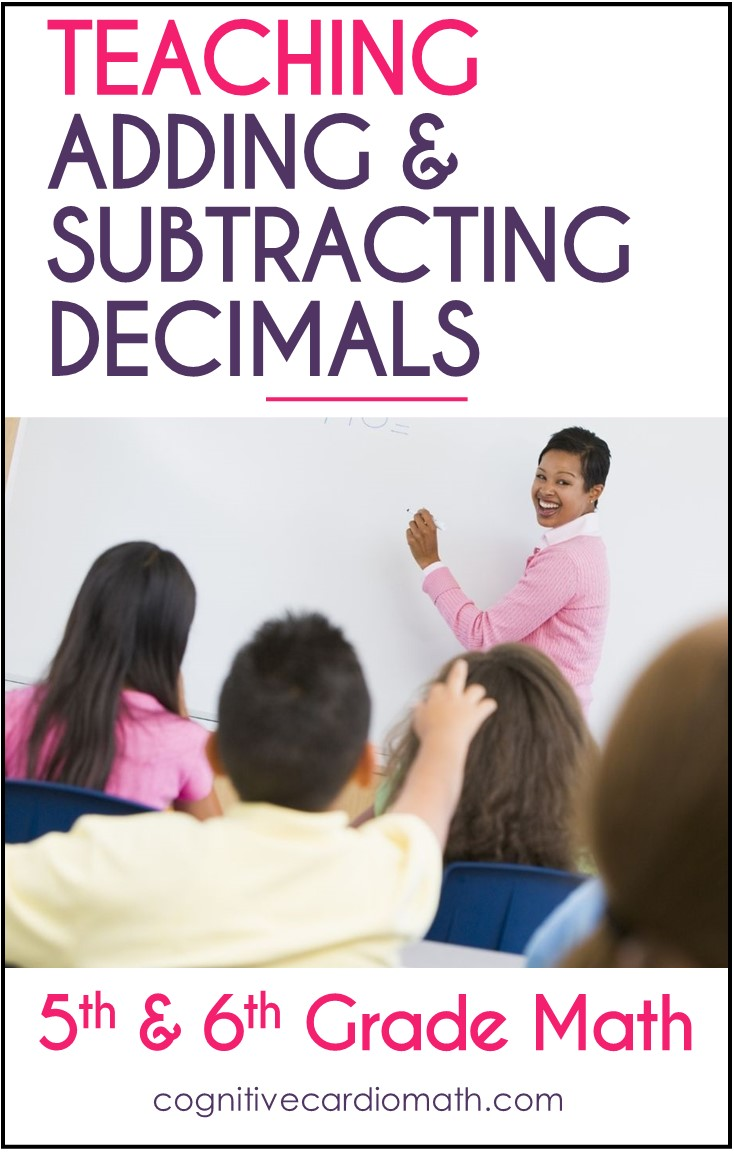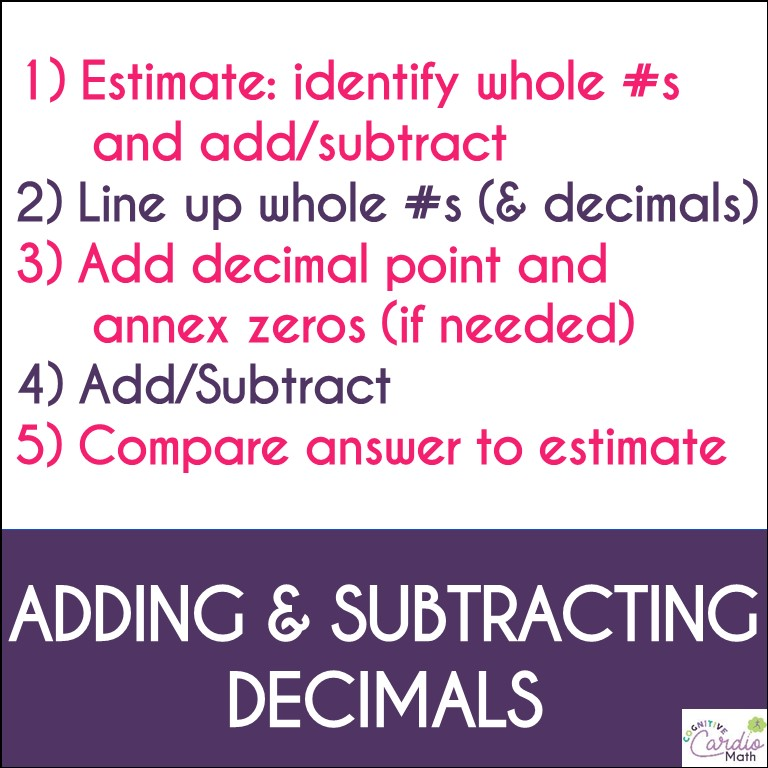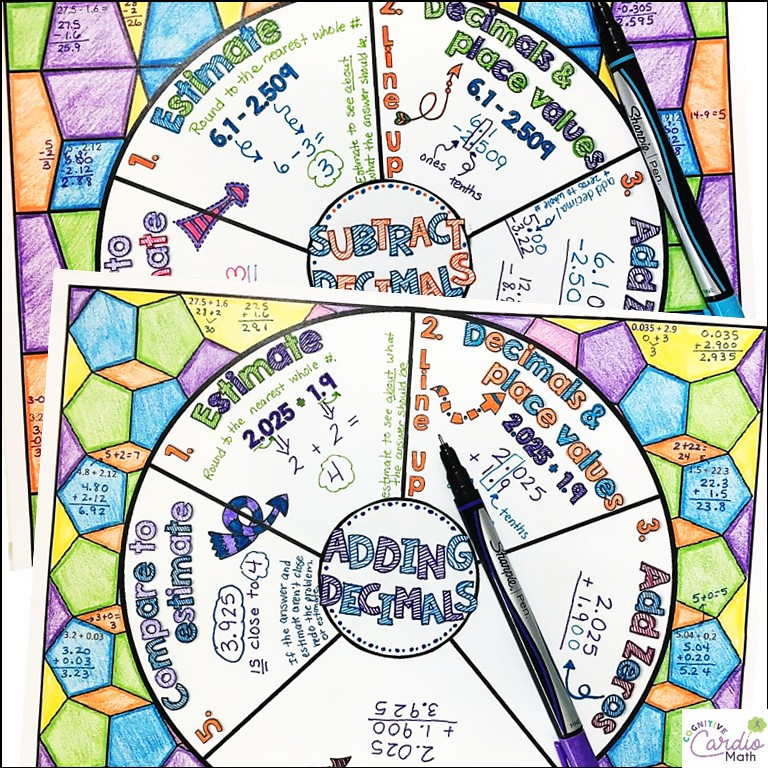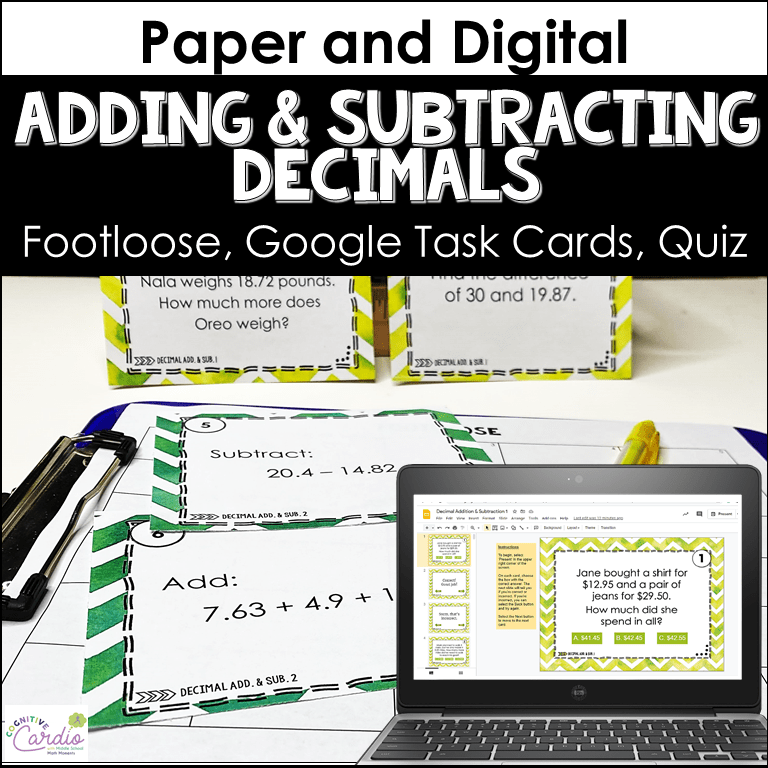## How to Teach Adding and Subtracting Decimals

How do you teach adding and subtracting decimals in upper elementary or middle school math?

In 6th grade math, my students have typically come to me knowing the ‘rules’ for adding and subtracting decimals.

However, when the number of digits in the numbers they’re adding or subtracting aren’t the same, they don’t necessarily line the numbers up the way they need to…even though they ‘know’ the rules.

Why is this?I believe it’s because they really don’t understand the point of ‘lining up the decimal points.’

My belief is reinforced by student comments I collected one year as we began our decimal operations unit.
I asked my 6th grade math students to solve 35.2 + 7.489 and then explain why their answer made sense. These are a few of their responses:

• “0.11009 makes sense because I tried my best and if I remember correctly, addition problems you don’t need to line the decimals together”
• “0.7838 makes sense because when I added I knew that it doesn’t matter how it’s lined up”
• “7.841 makes sense because with adding you only have to add the decimals on the top. Then you add and finally add the decimal back in.”
•  “79.41 makes sense because you do it just like an addition problem (that’s how I remember it anyway)”
• “42.689 – this makes sense to me because this is how I learned it. You do simple addition, but line up the decimal points”
• “42.689 makes sense because I used what my fifth grade teacher taught me, line up decimals, add zeros so everything is lined up and then solve.”
• “42.689 – I don’t know how it makes sense, but it’s how I learned to do it.”

Of the 120 students in my classes, only 8 said the answer made sense because “35 + 7 is 42,” or because “I estimated,” or even “when we’re doing addition, we know we end up with a bigger number.”

I don’t want to assume that students who didn’t write reasons like this didn’t think about those things at all, but for the majority of students, their answers “made sense” when they followed the rules – even if they didn’t remember the rules correctly.

## What's the Point?

What’s the purpose of lining up the decimal points?
Lining up the decimal points helps us line up the place values so that place values are added with or subtracted from ‘like’ place values.

• If students don’t understand the point of lining up the decimal points when adding and subtracting decimals, then somehow they’ve missed the idea of place value.

And what do they do if the numbers don’t even HAVE a decimal point?? (Some students get pretty lost when that happens.)

Because I believe students don’t understand the point of lining up the decimal point, I teach them to add and subtract decimals by doing the following:

1. Estimate: identify the whole numbers in the problem and then add or subtract them to get an estimate.

If necessary to help identify, students can circle the whole numbers.2. Line up the whole numbers according to their place value (ones place with ones place, tens with tens).

• If the whole numbers are lined up correctly, the decimals will automatically be lined up (and if students have a problem like 50 – 9.625, lining up the 0 and 9 in the ones places will put the decimal points in the correct place.

3. Add a decimal point if needed (for whole numbers) and annex zeros so the decimal part of the numbers have the same number of place values.

4. Complete the operation.

5. Compare the answer to the estimate to see if the answer is reasonable.

## Tools for Teaching Adding and Subtracting Decimals

One of my favorite ways to teach the addition and subtraction process is to use notes that emphasize estimating.

I include this in my and practice, and I also use math wheels for note-taking.The math wheels break the processes into the steps outlined above and allow room for examples and practice.

## Resources to Practice Adding and Subtracting Decimals

A couple practice decimal activities:
There are a couple free decimal activities found in the blog posts linked below. I’ve used these activities to give my students some additional decimal operations practice.

What are your tried and true methods for how to teach adding and subtracting decimals?## EllieWelcome to Cognitive Cardio Math! I’m Ellie, a wife, mom, grandma, and dog ‘mom,’ and I’ve spent just about my whole life in school! With nearly 30 years in education, I’ve taught:

• All subject areas in 4th and 5th grades
• Math, ELA, and science in 6th grade (middle school)

I’ve been creating resources for teachers since 2012 and have worked in the elearning industry for about five years as well!